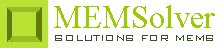PIZOTRAC,pcoef,wid,len,thc,volt,ymod,sel
Transverse piezoelectric actuator

pcoef     transverse piezoelectric coefficient in C/m2
wid        width of the piezoelectric film/element in µm
len         length of the piezoelectric film/element in µm
thc         thickness of the piezoelectric film/element in µm
volt       applied voltage in Volt
ymod    Young's modulus of the material in GPa
sel         number denoting the selected result.
Use 1 for actuator displacement, 2 for actuation force and 3 for electric field strength

Notes

Some materials generate an electric field when subjected to mechanical deformation or changes dimension when voltage is applied. This effect is called piezoelectric effect. Piezo actuators utilize the deformation of the piezoelectric material when an electric field is applied. The minute control in the actuator displacement and large mechanical load capacity possible in the case of piezoelectric actuators make them suitable in a variety of applications. Piezoelectric actuation does not consume dc power and also can provide both positive and negative force unlike capacitive actuation. On the flip side, piezoelectrically induced strains and displacement are small and requires large voltage to obtain a sufficient stroke.

When the applied electric field is perpendicular to the actuator displacement, the actuator is described to be in transverse or shear configuration. The transverse piezoelectric coefficient couples the orthogonal stress and electric field. Similar actuators are used in inkjet printer heads, ultrasonic surgical tools etc.

The actuator displacement and the generated force are proportional to the applied voltage for any given configuration. To get a larger displacement the length to thickness ratio of the piezoelectric film should be maximized.

The plot shows the actuator displacement for a transverse actuator for applied voltage ranging from -500V to +500V.

Assumptions

-The strain in the transducer is assumed to be uniform.
-The strains and electric fields in non-transverse directions are assumed to be zero.
-The piezoelectric element is free to deform.